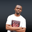Related Tags

euphoria
operators
communitycreator

# What are the operators in Euphoria?NDUKWE CHIDERA K.

### Introduction

An operator in Euphoria will perform an action between two or more operands on both sides. Euphoria Programming is a simple and flexible language that also provides a robust pool of operators to help developers achieve a lot. These operators are bundled into four major types, namely:

• Arithmetic operators
• Comparison operators
• Logical operators
• Assignment operators

### Arithmetic operators

These operators are used to carry out simple algebra and arithmetic computations. They are used to evaluate mathematical operations. They are listed and explained below. For the examples, let x be 10, and y be 30.

1. Addition operator (+): It adds two operand values, (x+y gives 40).

2. Subtraction operator (-): It subtracts the value of the right operand from the left, (y-x gives 20).

3. Division operator (/): It divides the operand on its left side with that on its right side. (y/x gives 3)

4. Multiplication operator (*): It multiplys both operands, (x*y gives 300).

5. Unary minus(-) and unary plus(+): They operate only on a single operand, the one that comes after it from the left to right direction. For example, (-y gives -30 while +x gives +10). They indicate if a value is positive(+) or negative (-).

### Comparison operator

These operators compare the operands at their left and right sides and then decide. Let’s look at the various comparison operators used in Euphoria:

1. Equal (=): It checks for equality between the operands’ left and right sides. If equality exists, it evaluates to true. For example, (10=30 gives false).

2. Not equal (!=): It checks if the operands are not equal in value and if not equal, it returns true. Otherwise, it returns false, (10!=30 gives true).

3. The greater than >: It compares the operand on its left with the right. It returns true if the left operand is greater in value than the right. Otherwise, it returns false. For example, (10>30 returns false).

4. The less than (<): It returns true if the value on the right side is less than the value on the left side. For example, (x<y returns true).

5. Less or equal to (<=): It checks if the value of the left operand is less than or equal to the value of the right operand, if yes then the condition becomes true, (10<=30 returns true).

6. Greater or equal to (>=): It checks if the value of the left operand is greater than or equal to the value of the right operand, if yes then the condition becomes true. If not, the condition evaluates false. For example,(10 >= 30 returns false).

### Logical Operators

These operators carryout out logic on operands in an expression and make a decision based on the output which is usually boolean.

1. Logical AND: It evaluates true if the two operands are none zero and false if otherwise.
2. Logical OR:It evaluates true if any of the operands have non-zero values and evaluates to false only if both operands are zero values.
3. Logical XOR: It checks the two operands and will return true only if any of them has a non-zero value. If both operands are zeros(that is false), or ones (true) then it evaluates to false.
4. NOT operator: It negates any value. It is actually a unary operator. For example, NOT Y, will make Y false, if it was originally true.

### Assignment operators

This set of operators assigns whatever is on its right to its left. This is true for the simple assignment operator(=). For example, name = "jane" this will automatically make name to have the value of jane.

Other not so simple assignment operators are also available. These are not so simple because they are combined with any of the arithmetic operators. This makes them evaluate the expression first before assigning the result to the left operand. These operators include:

• Addition and assignment operator (+=)
• Subtract and assignment operator(-=)
• Division and assignment operator(/=)
• Multiplication and assignment operator (*=)
• The concatenation and assignment operator (&=)

Note: The &= operator assigns to the left operand an ANDED value of the operand on the left and the one on the right. For example: X &= Y is the same as X = X & Y.

### Concatenation operator

Euphoria also has an operator for concatenation. The & operator is used to concatenate or join two objects. These joined objects will have the sum of the two joining objects as their length.

### Example

sequence x, y, z
x = {4, 5, 6}
y = {7}
z = {4, 5, 6} & {7}
print(1,z)


This produces the output: {4,5,6,7}

### Code

--declare the variables
sequence x, y, z
--assign values to the declared variables
x = {4, 5, 6}
y = {7}
--concatenate the x and y values
z = {4, 5, 6} & {7}
-- print the concatenated values
print(1,z)

In the above, we see the concatenation operator & is used to join the two sequences x and y to produce another sequence z. It is the same as the length of the two sequences joined together.

RELATED TAGS

euphoria
operators
communitycreator

CONTRIBUTORNDUKWE CHIDERA K.
RELATED COURSES

View all Courses

Keep Exploring

Learn in-demand tech skills in half the time